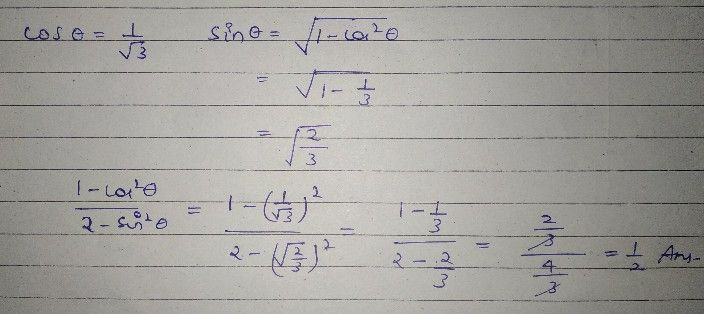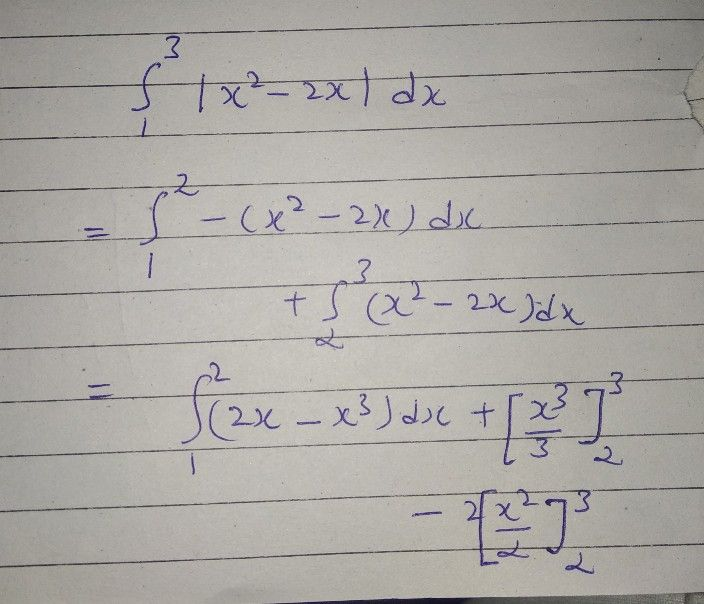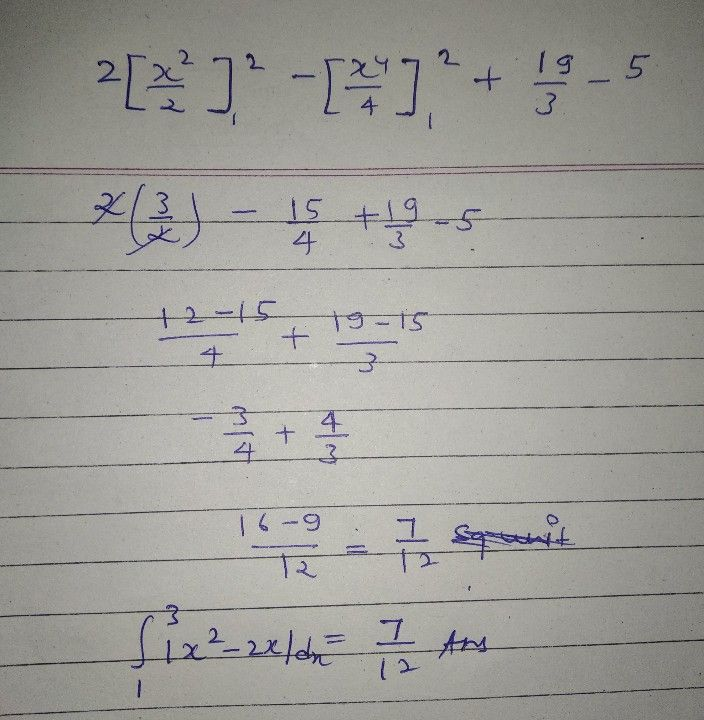Symbol
Problem$1$ If $cosθ=-$ $\sqrt{3}$ then evaluate $1-cos^{2}θ$ $3$ $-=-$ $2-sin^{2}θ$ $5$ $2.$ $3$ Evaluate $\int |x^{2}-2x|dx$ $1$
Calculus
Search count: 229
SolutionQanda teacher - RamanDear if you have any queries ask freely without any hesitation
please evaluate it Dear if you are satisfied ?
Please give 3000 coins as thank you gift this will not reduce your coins